# Class 10 Science: CBSE Sample Question Paper- Term I (2021-22) - 1 Notes | Study CBSE Sample Papers For Class 10 - Class 10

## Class 10: Class 10 Science: CBSE Sample Question Paper- Term I (2021-22) - 1 Notes | Study CBSE Sample Papers For Class 10 - Class 10

The document Class 10 Science: CBSE Sample Question Paper- Term I (2021-22) - 1 Notes | Study CBSE Sample Papers For Class 10 - Class 10 is a part of the Class 10 Course CBSE Sample Papers For Class 10.
All you need of Class 10 at this link: Class 10

Class-X
Time: 90 Minutes
M.M: 40

General Instructions:

1. The Question Paper contains three sections.
2. Section A has 24 questions. Attempt any 20 questions.
3. Section B has 24 questions. Attempt any 20 questions.
4. Section C has 12 questions. Attempt any 10 questions.
5. All questions carry equal marks.
6. There is no negative marking.
Section - A

Q.1: Choose the correct statement about the reaction taking place below:
6CO2 + 6H2O → C6H12O6 + 6O2
(a) It is an endothermic reaction.
(b) It is an exothermic reaction.
(c) It releases large amount of energy.
(d) All of these

The given reaction depicts the process of photosynthesis. Photosynthesis is an endothermic reaction that utilizes sunlight energy.

Q.2: Study the given equation: Na2CO+ XHCl → 2NaCl + CO2 + H2O
What will be the value of X to make it balanced equation?
(a) 1
(b) 2
(c) 3
(d) 4

To balance the given equation the number of atoms of each element should be same on both the sides. Hence, the ‘X’ value should be 2.

Na2CO3 + 2HCl 2NaCl + CO2 + H2O

Q.3: For a chemical reaction to take place:
(a) It must be accompanied with change in temperature and pressure.
(b) At least one of the reactants must be in a fixed quantity.
(c) It must follow the law of conservation of mass.
(d) All of the above

The balancing of a chemical equation is based on law of conservation of mass. This law states “mass can neither be created nor be destroyed in a chemical reaction”. This means that total mass of the elements in the reactant side of a chemical reaction should be equal to the total mass of the elements in the product side of a chemical reaction.

Q.4: The decomposition of silver chloride and silver bromide are carried out in the presence of:
(a) Electricity
(b) Sunlight
(c) Heat
(d) Water

The decomposition of silver chloride is a photochemical decomposition reaction. This type of decomposition reaction involves the use of light energy for decomposition.

Q.5: Choose the correct statement about the given reaction.
H2S + Cl2 → 2HCl + S
(i) Cl2 gets reduced to HCl.
(ii) H2S gets oxidized to S.
(iii) Cl2 is the oxidizing agent.
(iv) H2S is the oxidizing agent.
(a) I and II only
(b) II and III only
(c) I, II and III only
(d) All of these

H2S reduces Cl2 to HCl. H2S is the reducing agent.

Q.6: The aqueous solution of sodium acetate is
(a) Neutral
(b) Weakly Acidic
(c) Strongly Acidic
(d) Alkaline

Aqueous solution of sodium acetate is alkaline.

Q.7: Which of the following is a mineral acid?
(a) HCl
(b) H2SO4
(c) HNO3
(d) All of these

Acids prepared from the minerals of the earth are called mineral acids.  Examples include phosphoric acid, sulphuric acid, hydrochloric acid, nitric acid, etc.

Q.8: A  solution “X” gives pink colour with phenolphthalein. The pH is likely to be:
(a) 1
(b) 5
(c) 7
(d) 12

Phenolphthalein is often used as an indicator in acid–base titrations. For this application, it turns colorless in acidic solutions and pink in basic solutions and pH ranges above 7 are basic.

Q.9: In an experiment, Sonia found that an excess of dilute sulphuric acid reacts with both aqueous barium hydroxide and aqueous barium chloride respectively. In what way are the two reactions the same?
(a) A gas is evolved.
(b) An insoluble salt is formed
(c) Their final pH is 7
(d) A base is produced

In both the cases, precipitation occurs. A white precipitate of  BaSO4  is formed as shown below.

(i)  Ba(OH)2 + H2SO→ BaSO+ 2H2

(ii) BaCl2 + H2SO4 → BaSO4 + 2HCl

Q.10: Choose the correct equation for the metal which reacts vigorously with water.
(a) Ca(s) + 2H2O (l) → Ca(OH)2 (aq) + H2 (g)
(b) 2Na (s) + 2H2O (l) → 2NaOH (aq) + H2 (g)
(c) 2Al(s) + 3H2O(g) → Al2O3(s) + 3H2 (g)
(d) Mg(s)+ 2H2O(g) → Mg(OH) (aq)+ H2 (g)

Metals like sodium and potassium react violently with water, releasing huge amount of heat. So the reaction is highly exothermic. Hydrogen gas which is produced during the reaction catches fire.
2K(s) + 2H2O (l) → 2KOH (aq) + H2 (g) heat energy
2Na(s) + 2H2O (l) → 2NaOH (aq) + H2 (g) + heat energy

Q.11: The end product of fermentation in muscle cells is
(a) Lactic Acid
(b) Hydrochloric Acid
(c) Citric Acid
(d) Pyruvic Acid

Pyruvate is converted into lactic acid when there is a lack of oxygen in our muscle cells, which is also a three carbon molecule. During hard racing or exercise, the oxygen supply may not be enough for the needs of the muscle cells. When this happens, anaerobic respiration takes place, as well as aerobic respiration. The lactic acid that is formed by anaerobic respiration causes muscle fatigue and pain.

Q.12: Which of these statements is correct regarding the given figure of a heart.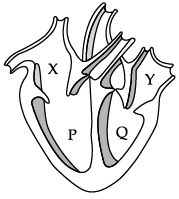(a) The blood from Y reaches the lungs.
(b) The blood from the body enters the heart through P.
(c) Y receives blood from the lungs.
(d) Q receives blood from the body.

In the given figure, X is right atrium. Right atrium receives deoxygenated blood from body. P is right ventricle which passes deoxygenated blood to lung for oxygenation. Y is left atrium that receives oxygenated blood from lungs. Q is left ventricle that supplies oxygenated blood to body.

Q.13: Haemoglobin has a maximum affinity for which of the following gases?
(a) Carbon Monoxide
(b) Carbon Dioxide
(c) Oxygen
(d) Nitrogen

Carbon monoxide CO binds with haemoglobin 200 times faster than even oxygen binds with haemoglobin. So haemoglobin has maximum affinity for CO. Moreover, haemoglobin combines with carbon monoxide to form carboxyhaemoglobin. So carbon monoxide reduces oxygen carrying capacity of haemoglobin by amount equivalent to amount of haemoglobin converted to carboxyhaemoglobin.

Q.14: The metal __________  is associated with haemoglobin.
(a) Copper
(b) Iron
(c) Aluminium
(d) Potassium

Iron is an essential element for blood production. About 70 percent of your body’s iron is found in the red blood cells of your blood called hemoglobin and in muscle cells called myoglobin. Hemoglobin is essential for transferring oxygen in your blood from the lungs to the tissues.

Q.15: Which of these statements is correct about transpiration?
(i) It causes absorption of mineral salts.
(ii) It regulates plant temperature.
(iii) It causes wilting and injury in plants.
(iv) It causes an ascent of sap.
(a) I and IV only
(b) I, II and III only
(c) I, II and IV only
(d) All of these

Transpirational pull created by transpiration of water from leaf surface causes ascent of sap (upward movement of water and mineral salts). The sap absorbed from the soil contains low concentration of mineral salts. The loss of water through transpiration increases the concentration of mineral salts in the plant. Due to the heat of the Sun, temperature of the plant increases which may be harmful for the plants. Transpiration lowers down the temperature of the plant by 10°−15°C. Due to transpiration being higher than the rate of water absorption at noon time wilting or loss of turgidity is quite common. Excess dehydration reduces the rate of photosynthesis and other metabolic activities, which may further lead to deformation in plants.

Q.16: Which of these represent the correct pathway of carbon dioxide after the exchange of gases in the lungs?
(a) Alveolus  →  bronchiole  →  bronchus →  trachea →  nose
(b) Alveolus  →  bronchus  →  bronchiole →  trachea →  nose
(c) Trachea  →  bronchus →  bronchiole →  alveolus →  nose
(d) Trachea  →  bronchiole  →  bronchus →  alveolus →  nose

The correct pathway of air that leaves the lungs is: Alveolus bronchiole bronchus trachea nose

Q.17: _____ mirrors can be used to burn a piece of paper using the reflected rays of the sun.
(a) A plane mirror
(b) A concave mirror
(c) A convex mirror
(d) All of the above

A concave mirror can be used to burn a piece of a paper by focusing reflected rays of the sun.

Q.18: What is the image formed when a convergent beam of light is incident on a plane mirror?
(a) Upright and Real
(b) Upright and Virtual
(c) Inverted and Virtual
(d) Inverted and Real

The image formed by plane mirror is always virtual and erect. It is laterally inverted i.e., the right side of the object appears left side of the image and vice versa.

Q.19: Read the given statements carefully.
(i) A light wave enters from the first medium into the second medium.
(ii) Its velocity in the second medium is double that in the first medium.
For the phenomenon of total internal reflection to take place, the angle of incidence must be greater than a certain value. This value is _________.
(a) 90°
(b) 60°
(c) 45°
(d) 30°

Given, velocity of light wave doubles in second medium. Let velocity of light in first medium be v
∴ Velocity of light in second medium = 2v
sin C = Velocity in 1st medium/Velocity in 2nd medium
| ∴ v/2v = 1/2| ⇒ C = 30°

Q.20: Under which of these cases, you will find an inverted image in a convex mirror?
(a) Under no circumstances
(b) When the object is very far from the mirror
(c) When the object is at a distance equal to the radius of curvature of the mirror
(d) When the distance of the object from the mirror is equal to the focal length of the mirror

Only a concave mirror can be used to produce an inverted image, which too when an object is located at a position of more than one focal length from the concave mirror.

Q.21: When an object is at infinity from a concave mirror, then the image formed is __________.
(i) At the focus
(ii) Virtual and erect
(iii) Highly enlarged
(iv). Real and inverted
(a) I and IV only
(b) I, and II only
(c) I, II, III only
(d) I, III, IV only

When the object is at infinity, the incident rays are parallel to the principal axis. Hence the image is formed at the focus. And the image is real and inverted but in diminished size. Hence, we can say that the image formed at the focus.

Q.22: A converging lens has a focal length of 15 cm. If an object is placed 10 cm from the optical centre of the lens, the characteristics of the image formed will be:
(a) Real, inverted and diminished
(b) Real, inverted and of the same size as the object
(c) Real, inverted and enlarged
(d) Virtual, upright and enlarged

By using the lens equation, 1/u + 1/v = 1/f

1/15 = 1/10 + 1/v

Hence, v = -30 cm

Now, we know that, m=v/u

Therefore;

m = -30/10 = -3 Since the answer is in "-", the image is inverted and enlarged.

Also, as the image formed is on the same side as that of the object, the image formed is real.

The correct answer is Real, Inverted and enlarged. (c)

Q.23: A real and inverted image of the same size is formed by a convex lens, when an object is placed ___________.
(a) At infinity
(b) At the focus of the lens
(c) At twice its focal length
(d) Between f and the lens

To obtain a real, inverted image of the same size, the object must be placed at 2f or twice the focal length of the convex lens.

Q.24: What is the frequency of yellow light of wavelength 550 nm, if the speed of light is 3 × 108 m/s?
(a) 5.4  × 1014 Hz
(b) 3.4 × 1012 Hz
(c) 3.0 × 1014 Hz
(d) 2.8 × 1012 Hz

Given,
Wavelength λ = 550 nm
= 550 × 10–9m
Speed of light, C = 3 × 108 m/s
We know that
Frequency = Speed of light/Wavelength
Frequency = (3x108)/(550x10-9)
Frequency = 5.4 × 1014 Hz

Section-B

Q.25: Marble’s popularity began in ancient Rome and Greece, where white and off-white marble were used to construct a variety of structures, from hand-held sculptures to massive pillars and buildings.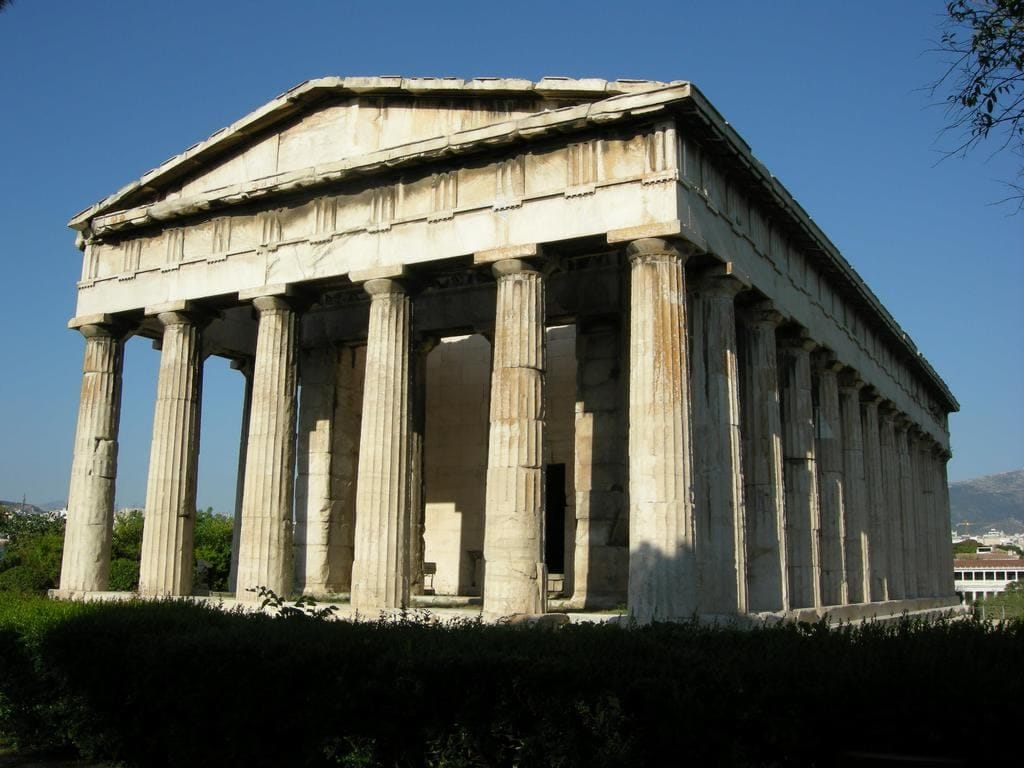The substance not likely to contain CaCO3 is
(a) Dolomite
(b) A marble statue
(c) Calcined Gypsum
(d) Sea Shells.

The composition of gypsum is CaSO4 ∙2H2O. It does not have CaCO3.

Q.26: A student added 10g of calcium carbonate in a rigid container, secured it tightly and started to heat it. After some time, an increase in pressure was observed, the pressure reading was then noted at intervals of 5 minutes and plotted against time, in a graph as shown below. During which time interval did maximum decomposition take place?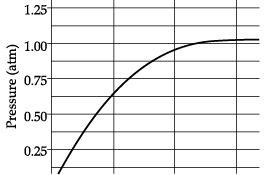(a) 15-20 min
(b) 10-15 min
(c) 5-10 min
(d) 0-5 min

The maximum decomposition is when the pressure is maximum. As we can see in graph that from 0 to 5 minutes, the pressure increases from 0 to 0.625 atm.

Q.27: Gas A, obtained above is a reactant for a very important biochemical process that occurs in the presence of sunlight. Identify the name of the process -
(a) Respiration
(b) Photosynthesis
(c) Transpiration
(d) Photolysis

When CaCO3 is heated, the following reaction takes place:
CaCO3 → CaO + CO2
The gas evolved  is carbon dioxide, which is utilised in the process of photosynthesis.

Q.28: Marble statues are corroded or stained rainwater. Identify the main reason.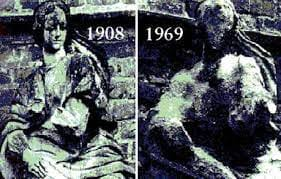(a) Decomposition of calcium carbonate to calcium oxide
(b) Polluted water is basic in nature hence it reacts with calcium carbonate
(c) Polluted water is acidic in nature hence it reacts with calcium carbonate
(d) Calcium carbonate dissolves in water to give calcium hydroxide.

Chemically, marble is Calcium Carbonate.
The atmosphere contains many oxides, which dissolve in water forming acids like sulphuric nitric which are common due to modern pollution. Even carbon dioxide forms carbonic acid which also does damage.
These will react with marble and result in formation of calcium salt, carbon dioxide and water. So, under extended periods, the wear of marble statues is expected.

Q.29: Calcium oxide can be reduced to calcium, by heating with sodium metal. Which compound would act as an oxidizing agent in the above process?
(a) Sodium
(b) Sodium oxide
(c) Calcium
(d) Calcium oxide

A substance that undergoes reduction is an oxidizing agent. Here, CaO is losing oxygen and undergoing reduction. So, CaO is the oxidizing agent.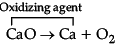Q.30: Sodium hydrogen carbonate when added to acetic acid evolves a gas. Which of the following statements are true about the gas evolved?
(i) It turns lime water milky
(ii) It extinguishes a burning splinter
(iii) It dissolves in a solution of sodium hydroxide
(iv) It has a pungent odour
(a) (i) and (ii)
(b) (i), (ii) and (iii)
(c) (ii), (iii) and (iv)
(d) (i) and (iv)

Sodium hydrogen carbonate when reacts with acetic acid evolves carbon dioxide gas. Carbon dioxide gas turns lime water milky, extinguishes fire on a burning splinter and dissolves in a solution of sodium ethanoate.

Q.31: Directions: The consist of two statements. Assertion (A) and Reason (R). Answer these questions selecting the appropriate option given below:
Assertion: When an electric current is passed through water, it decomposes to give oxygen and hydrogen. This type of reaction is called electrolysis.
Reason : This reaction involves the use of electricity for the decomposition of the reactant molecules.
(a) Both A and R are true and R is the correct explanation of A.
(b) Both A and R are true but R is NOT the correct explanation of A.
(c) A is true but R is false.
(d) A is false and R is true.

Electrolysis (Electrolytic decomposition) involves the use of electricity for the decomposition of the reactant molecules. When electric current is passed through water, it decomposes to give oxygen and hydrogen.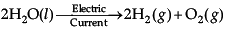Q.32: Directions: The consist of two statements. Assertion (A) and Reason (R). Answer these questions selecting the appropriate option given below:
Assertion:  An aqueous solution of acid conducts electricity.
Reason:  An acid in the aqueous solution ionizes to produce H+ ions and the corresponding negative ions.
(a) Both A and R are true and R is the correct explanation of A.
(b) Both A and R are true but R is NOT the correct explanation of A.
(c) A is true but R is false.
(d) A is false and R is true.

An acid in the aqueous solution ionizes to produce H+ ions and the corresponding negative ions. For example, HCl gives H+ and Cl ions and HNOgives H+ and NO3 ions. Due to the presence of ions in the solution, it conducts electricity.

Q.33: Directions: The consist of two statements. Assertion (A) and Reason (R). Answer these questions selecting the appropriate option given below:
Assertion: Based on the given experimental setup, it can be concluded that exhaled air contains more carbon dioxide than inhaled air.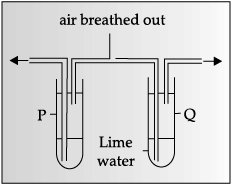Reason: Carbon dioxide gas turns lime water milky.

(a) Both A and R are true and R is the correct explanation of A.
(b) Both A and R are true but R is NOT the correct explanation of A.
(c) A is true but R is false.
(d) A is false and R is true.

Exhaled air contains more carbon dioxide than inhaled air, which converts lime water milky.

Q.34: Directions: The consist of two statements. Assertion (A) and Reason (R). Answer these questions selecting the appropriate option given below:
Assertion: The focal length of a convex mirror increases when it is held underwater.
Reason: The focal length of a concave mirror is independent of the medium in which it is placed.

(a) Both A and R are true and R is the correct explanation of A.
(b) Both A and R are true but R is NOT the correct explanation of A.
(c) A is true but R is false.
(d) A is false and R is true.

Focal length is the property of a mirror. It is always independent of the medium in which it is placed.

Q.35: Identify the correct representation of reaction occurring during chloralkali process
(A) 2NaCl(l) + 2H2O(l) → 2NaOH(l) + Cl2(g) + H2(g)
(B) 2NaCl(aq) + 2H2O(aq) → 2NaOH(aq) + Cl2(g) + H2(g)
(C) 2NaCl(aq) + 2H2O(l) → 2NaOH(aq) + Cl2(aq) + H2(aq)
(D) 2NaCl(aq) + 2H2O(l) → 2NaOH(aq) + Cl2(g) + H2(g)

When electricity is passed through an aqueous solution of sodium chloride (called brine), it decomposes to form sodium hydroxide. The process is called the chloralkaline process because of the products formed–chlor for chlorine and alkali for sodium hydroxide.

Q.36: What happens when calcium is treated with water?
(i) It does not react with water
(ii) It reacts violently with water
(iii) It reacts less violently with water
(iv) Bubbles of hydrogen gas formed stick to the surface of calcium
(a) (i) and (iv)
(b) (ii) and (iii)
(c) (i) and (ii)
(d) (iii) and (iv)

Calcium reacts slowly with water. The reaction forms calcium hydroxide, Ca(OH)2 and hydrogen gas (H2). The calcium metal sinks in water and after an hour or so bubbles of hydrogen are observed, stuck to the surface of the metal.

Q.37: The correct sequence of circulation of blood in the human body is :
(A) Lungs → Pulmonary vein → Left ventricle → Left atrium → Aorta → Body Organs → Vena Cava → Right Ventricle → Right atrium → Pulmonary artery  → Lungs
(B) Lungs → Pulmonary vein → Left atrium → Left ventricle → Aorta → Body organs → Vena cava → Right atrium → Right ventricle → Pulmonary artery → Lungs.
(C) Lungs → Left atrium → Left ventricle → Body organs  → Vena cava → Right atrium → Right ventricle  → Pulmonary artery → Pulmonary vein → Aorta → Lungs.
(D) Lungs → Pulmonary artery → Pulmonary vein → Body organs → Vena cava → Right atrium → Right ventricle → Aorta → Left ventricle → Left atrium  → Lungs

Lungs supply oxygen rich blood to the left atrium of the heart. The left upper chamber (left atrium) then relaxes. Gradually, it contracts and the blood is allowed to enter the next chamber (left ventricle), as it expands. When the muscular left lower chamber of heart contracts the blood is pumped out to the body via aorta. Deoxygenated blood reaches from the body to the upper chamber on the right side of heart right atrium and it expands. As this part contracts, the corresponding lower chamber (right ventricle) dilates. This transfers the blood to right ventricle, which in turn pumps it to the lungs for oxygenation.

Q.38: Which of these statements is correct about peristaltic movement?
(A) It is the contraction and expansion of lungs.
(B) It is the contraction and expansion of walls of food pipe.
(C) It is the movement of limbs while walking.
(D) It is the movement of blood throughout the body.

The wall of alimentary canal contains muscle layers. Rhythmic contraction and relaxation of these muscles pushes the food forward. This is called peristalsis, which occurs all along the gut. This is a kind of movement which facilitates the movement of food inside the alimentary canal.

Q.39: In the given experiment set-up,  _______  gas  is evolved as gas bubbles in the test tube.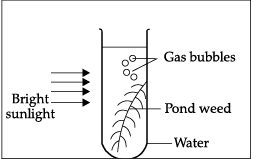(A) Oxygen
(B) Carbon dioxide
(C) Nitrogen
(D) Hydrogen

Oxygen is evolved during the process of photosynthesis.

Q.40: The blood sample _____  is most probably from a pulmonary artery.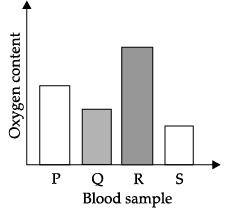(A) P
(B) Q
(C) R
(D) S

Arteries carry blood from the heart to various organs of the body. They generally carry oxygen rich blood except for pulmonary artery. Pulmonary artery is the only artery that carries carbon dioxide rich blood from heart to lungs.

Q.41: Identify Y and Z in the given flow chart.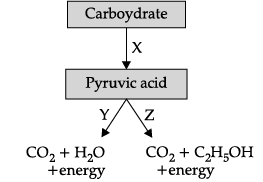(a) Y- Aerobic, Z- Anaerobic
(b) Y –Anaerobic, Z- Aerobic
(c) Y-Glycolysis, Z- Fermentation
(d) Y- Glycolysis, Z – Aerobic

In the given flow chart X represents glycolysis, Y is aerobic and Z is anaerobic respiration.

Q.42: A 10 mm long awl pin is placed vertically in front of a concave mirror. A 5 mm long image of the awl pin is formed at 30 cm in front of the mirror. The focal length of this mirror is
(a) – 30 cm
(b) – 20 cm
(c) – 40 cm
(d) – 60 cm

Here the size of object
h = 10 mm = 1 cm Size of the image h’ = 5 mm = 0.5 cm
Distance of image from the pole of mirror v = – 30 cm
Let distance of object = u
Let focal length = f
Using mirror formula 1/v + 1/u = 1/f
Therefore, the value of u is needed for that magnification formula will be used.
Magnification (m) = h'/h = -v/u
1/f = 1/v + 1/u
Putting all the values in mirror formula :
(1/(-30)) + (1/(-60)) = 1/f
⇒ ((2+1)/-60) = 1/f
1/f = 3/(-60) ⇒ f = -20 cm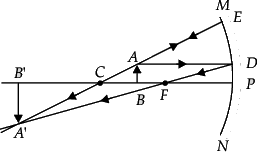Q.43: A child is standing in front of a magic mirror. She finds the image of her head bigger, the middle portion of her body of the same size and that of the legs smaller. The following is the order of combinations for the magic mirror from the top.
(a) Plane, convex, and concave
(b) Convex, concave, and plane
(c) Concave, plane, and convex
(d) Convex, plane, and concave

When electricity is passed through an aqueous solution of sodium chloride (called brine), it decomposes to form sodium hydroxide. The process is called the chloralkaline process because of the products formed–chlor for chlorine and alkali for sodium hydroxide.

Q.44: At which position, the object is needed to be placed to produce an image by a convex lens, at the position shown in the figure?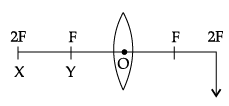(a) Between Y and O
(b) Between X and Y
(c) At Y
(d) At X

The object is needed to be placed at X (2F) to obtain an image by a convex lens. If the image is to be produced at 2F, in case of a convex lens, then the object needs to be placed at X (2F).

Q.45: The correct graphical relation between sine of angle of incidence (i) and sine of angle of refraction (r) for a given medium is :
(a)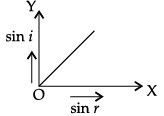(b)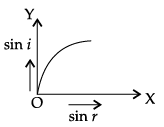(c)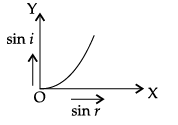(d)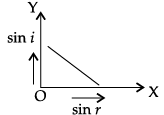According to Snell’s law,
sin i/ sin r = μ
This means sin i ∝ sin r. For a given medium μ is constant. Thus, sin i increases linearly with sin r.

Q.46: Choose the correct diagram that depicts the reflection of a ray of light on a spherical mirror?
(a)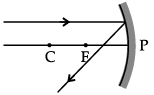(b)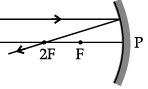(c)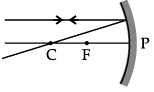(d)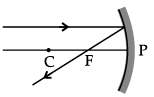A ray parallel to the principal axis, after reflection, will pass through the principal focus in case of a concave mirror or appear to diverge from the principal focus in case of a convex mirror.

Q.47: Vivaan placed an object O at the position shown in the given figure.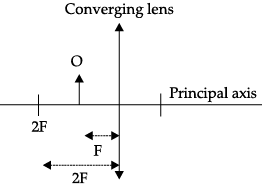The characteristics of the image formed are:
(a) Real, inverted and diminished
(b) Real, inverted and enlarged
(c) Virtual, upright and enlarged
(d) No image is formed

The correct emergent ray is E2. Emergent ray is the refracted ray which is emerged out of a medium after refraction.

Q.48: In the given diagram, an incident ray strikes a rectangular glass block.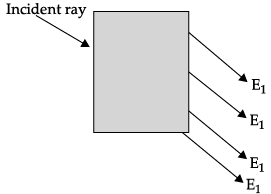The correct emergent ray is :
(a) E1
(b) E2
(c) E3
(d) E
4

The correct emergent ray is E2. Emergent ray is the refracted ray which is emerged out of a medium after refraction.

Section-C

Q.49: Case:- The Salt Story
From: The New Indian Express 9 March 2021
The salt pans in Marakkanam, a port town about 120 km from Chennai are the third largest producer of salt in Tamil Nadu. Separation of salt from water is a laborious process and the salt obtained is used as raw materials for manufacture of various sodium compounds. One such compound is Sodium hydrogen carbonate, used in baking, as an antacid and in soda acid fire extinguishers. The table shows the mass of various compounds obtained when 1litre of sea water is evaporated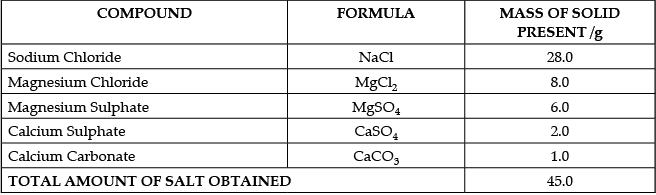Which compound in the table reacts with acids to release carbon dioxide?
(a) NaCl
(b) CaSO4
(c) CaCO3
(d) MgSO
4

We know that

• Acids react with Metal Carbonates and Hydrogen Carbonates to form salt, carbon dioxide and water.
• Hence from the options, Acid will react with Calcium Carbonate (CaCO3) to release Carbon Dioxide
• An example of the reaction is Calcium carbonate reacts with hydrochloric acid to form carbon dioxide gas.

Q.50: Case:- The Salt Story
From: The New Indian Express 9 March 2021
The salt pans in Marakkanam, a port town about 120 km from Chennai are the third largest producer of salt in Tamil Nadu. Separation of salt from water is a laborious process and the salt obtained is used as raw materials for manufacture of various sodium compounds. One such compound is Sodium hydrogen carbonate, used in baking, as an antacid and in soda acid fire extinguishers. The table shows the mass of various compounds obtained when 1litre of sea water is evaporatedHow many grams of Magnesium Sulphate are present in 135g of solid left by evaporation of sea water?
(a) 6g
(b) 12g
(c) 18g
(d) 24g

In 45 g solid, we have 6g Magnesium Sulphate

In 1 g solid, we have 6/45 g Magnesium Sulphate

In 135 g solid, we have 6/45 × 135 g = 6 × 3 = 18g  Magnesium Sulphate

Q.51: Case:- The Salt Story
From: The New Indian Express 9 March 2021
The salt pans in Marakkanam, a port town about 120 km from Chennai are the third largest producer of salt in Tamil Nadu. Separation of salt from water is a laborious process and the salt obtained is used as raw materials for manufacture of various sodium compounds. One such compound is Sodium hydrogen carbonate, used in baking, as an antacid and in soda acid fire extinguishers. The table shows the mass of various compounds obtained when 1litre of sea water is evaporatedWhat is the saturated solution of Sodium Chloride called?
(a) Brine
(b) Lime water
(c) Slaked lime
(d) Soda water

Brine is salt water - which is a highly concentrated water solution of common salt (sodium chloride).

Q.52: Case:- The Salt Story
From: The New Indian Express 9 March 2021
The salt pans in Marakkanam, a port town about 120 km from Chennai are the third largest producer of salt in Tamil Nadu. Separation of salt from water is a laborious process and the salt obtained is used as raw materials for manufacture of various sodium compounds. One such compound is Sodium hydrogen carbonate, used in baking, as an antacid and in soda acid fire extinguishers. The table shows the mass of various compounds obtained when 1litre of sea water is evaporatedWhat is the pH of the acid which is used in the formation of common salt?
(a) Between 1 to 3
(b) Between 6 to 8
(c) Between 8 to 10
(d) Between 11 to 13

To make salt neutral, we need strong acid and strong base to make salt. So, for the formation of common salt the pH of the acid is between 1 to 3.

Q.53: The Figure shown below represents an activity to prove the requirements for photosynthesis. During this activity, two healthy potted plants were kept in the dark for 72 hours. After 72 hours, KOH is kept in the watch glass in setup X and not in setup Y. Both these setups are air tight and have been kept in light for 6 hours. Then, Iodine Test is performed with one leaf from each of the two plants X and Y.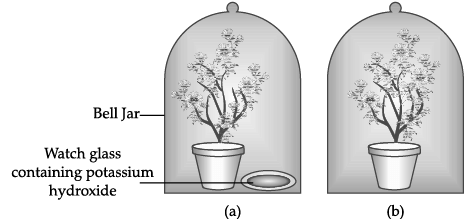This experimental set up is used to prove essentiality of which of the following requirements of photosynthesis?
(a) Chlorophyll
(b) Oxygen
(c) Carbon dioxide
(d) Sunlight

To perform photosynthesis, plants need three things: carbon dioxide, water, and sunlight.

In the experiment the plants are kept airtight with the presence of potassium hydroxide which absorbs all the carbon dioxide present in the jar, to prove the essentiality of carbon dioxide.

Thus, this experiment is used to prove essentiality of Carbon Dioxide.

Q.54: The Figure shown below represents an activity to prove the requirements for photosynthesis. During this activity, two healthy potted plants were kept in the dark for 72 hours. After 72 hours, KOH is kept in the watch glass in setup X and not in setup Y. Both these setups are air tight and have been kept in light for 6 hours. Then, Iodine Test is performed with one leaf from each of the two plants X and Y.The function of KOH is to absorb
(a) Oxygen.
(b) Carbon dioxide.
(c) Moisture.
(d) Sunlight.

The potassium hydroxide absorbs the carbon dioxide liberating in the reaction and forms the potassium carbonate K2CO3.

Q.55: The Figure shown below represents an activity to prove the requirements for photosynthesis. During this activity, two healthy potted plants were kept in the dark for 72 hours. After 72 hours, KOH is kept in the watch glass in setup X and not in setup Y. Both these setups are air tight and have been kept in light for 6 hours. Then, Iodine Test is performed with one leaf from each of the two plants X and Y.Which of the following statements shows the correct results of Iodine Test performed on the leaf from plant X and Y respectively?
(a) Blue - black colour would be obtained on the leaf of plant X and no change in colour on leaf of plant Y.
(b) Blue - black colour would be obtained on the leaf of plant Y and no change in colour on leaf of plant X.
(c) Red colour would be obtained on the leaf of plant X and brown colour on the leaf of plant Y.
(d) Red colour would be obtained on the leaf of plant Y and brown colour on the leaf of plant X.

An Iodine test is used to detect presence of starch in the leaf

• If putting Iodine solution turns the leaf into blue-black, it means starch is present
• If there is no blue-black colour, no starch is present
• In Plant X does not have Carbon Dioxide, so starch will not be present. Thus, it will not turn blue black
• In Plant Y, since there is Carbon Dioxide, so starch is present. Thus, it will turn blue black

Q.56: The Figure shown below represents an activity to prove the requirements for photosynthesis. During this activity, two healthy potted plants were kept in the dark for 72 hours. After 72 hours, KOH is kept in the watch glass in setup X and not in setup Y. Both these setups are air tight and have been kept in light for 6 hours. Then, Iodine Test is performed with one leaf from each of the two plants X and Y.Which of the following steps can be followed for making the apparatus air tight?
i. placing the plants on glass plate
ii. using a suction pump.
iii. applying vaseline to seal the bottom of jar.
iv. creating vacuum
(a) i and ii
(b) ii. and iii
(c) i. and iii
(d) ii. and iv

Let’s check each option

• For (i) - If we place plants on glass plate, it will make sure that there is no gap between the jar and the bottom. So, we can use this step.
• For (ii) - If we use a suction pump, it will remove all the air inside the jar. So, this step does not make the apparatus air tight, it just removes the air.

• For (iii) - Vaseline will seal moisture and help the jar set at the bottom. So, we can use this step.

• For (iv) - If we create vaccum, it will remove all the air inside the jar. So, this step does not make the apparatus air tight, it just removes the air.

Thus, (i) and (iii) are correct

Q.57: Noor, a young student, was trying to demonstrate some properties of light in her Science project work. She kept ‘X’ inside the box (as shown in the figure) and with the help of a laser pointer made light rays pass through the holes on one side of the box. She had a small butter-paper screen to see the spots of light being cast as they emerged.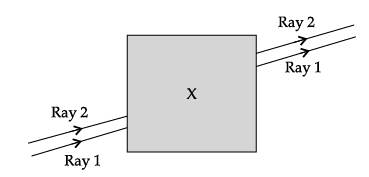What could be the ‘X’ that she placed inside the box to make the rays behave as shown?
(a) a converging lens
(b) a parallel-sided glass block
(c) a plane mirror
(d) a triangular prism

a parallel-sided glass block

Q.58: Noor, a young student, was trying to demonstrate some properties of light in her Science project work. She kept ‘X’ inside the box (as shown in the figure) and with the help of a laser pointer made light rays pass through the holes on one side of the box. She had a small butter-paper screen to see the spots of light being cast as they emerged.She measured the angles of incidence for both the rays on the left side of the box to be 48.6°. She knew the refractive index of the material ‘X’ inside the box was 1.5. What will be the approximate value of angle of refraction?
(use the value: sin 48.6° ≈ 0.75)
(a) 45°
(b) 40°
(c) 30°
(d) 60°

Refractive index of medium = sin i/sin r

1.5 = sin 48.6°/sinr
1.5 = 0.75/sin r
sin r = 0.75/0.5
sin r = 0.5
r = sin−1(0.5)
r = 30°

Q.59: Noor, a young student, was trying to demonstrate some properties of light in her Science project work. She kept ‘X’ inside the box (as shown in the figure) and with the help of a laser pointer made light rays pass through the holes on one side of the box. She had a small butter-paper screen to see the spots of light being cast as they emerged.Her friend noted the following observations from this demonstration:
i. Glass is optically rarer than air.
ii. Air and glass allow light to pass through them with the same velocity.
iii. Air is optically rarer than glass.
iv. Speed of light through a denser medium is faster than that of a rarer medium.
v. The ratio: sin of angle of incidence in the first medium to the ratio of sin of angle of refraction in the second medium, gives the refractive index of the second material with respect to the first one.
Which one of the combination of the above statements given below is correct.

(a) ii, iv and v are correct.
(b) iii and iv are correct.
(c) i, iv and v are correct.
(d) iii and v are correct.

Let’s look at each options

• For (i) - A medium is rarer when the speed of light increases when light travels through that medium. Since Speed of light in air is more than the speed of light in glass, which means air is optically rarer than glass. So, option (i) is incorrect.
• For (ii) - Actually, Speed of Light in Air is more than Speed of Light in Glass. Thus, they have different velocities. So, option (ii) is also incorrect .

• For (iii) - We discussed in part (i) that Air is optically rarer than glass. Thus, option (iii) is correct.

• For (iv) - Speed of light through a denser medium is slower than that of a rarer medium. So, option (iv) is also incorrect.

• For (v) - The ratio of sin of angle of incidence in the first medium to the ratio of sin of angle of refraction in the second medium, gives the refractive index of the second material with respect to the first one. Thus, option (v) is correct.

Thus, options (iii) and (v) are correct.

Q.60: Noor, a young student, was trying to demonstrate some properties of light in her Science project work. She kept ‘X’ inside the box (as shown in the figure) and with the help of a laser pointer made light rays pass through the holes on one side of the box. She had a small butter-paper screen to see the spots of light being cast as they emerged.If the object inside the box was made of a material with a refractive index less than 1.5 then the

(a) lateral shift of the rays would have been less.
(b) lateral shift of the rays would have been more.
(c) lateral shift of the rays would remain the same as before.
(d) there is not enough information to comment on any of the above statements

Now,

• Greater the refractive index, smaller the angle of refraction
• If we decrease the refractive index, the angle of refraction increases

• This lateral shift of rays will be less, shift will be less because of angle of refraction is becoming equal to angle of incidence

The document Class 10 Science: CBSE Sample Question Paper- Term I (2021-22) - 1 Notes | Study CBSE Sample Papers For Class 10 - Class 10 is a part of the Class 10 Course CBSE Sample Papers For Class 10.
All you need of Class 10 at this link: Class 10Use Code STAYHOME200 and get INR 200 additional OFF

## CBSE Sample Papers For Class 10

174 docs|7 tests

Track your progress, build streaks, highlight & save important lessons and more!

,

,

,

,

,

,

,

,

,

,

,

,

,

,

,

,

,

,

,

,

,

;